# Using Graphical Objects in Python

Dr McFadden has 20+ years of experience in IT and over 12 years in teaching college courses across multiple colleges, both in person and online.

This lesson discusses Python's graphical objects. You will learn how to use GraphWin, Point, Circle, Oval, Line, Text, and Rectangle objects in a Python GUI.

## GraphWin

GraphWin is a class in graphics.py (GNU open-source software) written by John Zelle for a textbook on Python. It is meant to make it easier to play with writing Python graphics code. The underlying Python graphics package that it uses is Tkinter.

To use GraphWin, you need to download and import graphics.py and make sure you have Tkinter. Then to create a graphics window you call the constructor passing the title, width and height. For example, to create a graphics window with title 'My Graphics' of size 250 by 250 we code:

`from graphics import *def main():    win = GraphWin(''My Graphics'', 250, 250) # specifies graphics window size 250x250    win.getMouse() # keep window up    win.close()main()`

We can also make changes to the window such as set the background color:

win.setBackground("red")

### Point

A Point object is represented by the x and y coordinates and draws a point. To draw a point in the middle of the graphics window we can implement:

`from graphics import *def main():    win = GraphWin(''My Graphics'', 250, 250) # specifies graphics window size 250x250    g = Point(125, 125) # creates a Point object at x=125 y=125    g.draw(win) # draws to the graphics window    win.getMouse() # keep window up    win.close()main()`

In this code we create a Point object placing it at coordinates x=125 and y=125, which is the middle of the graphics window, and then we draw it.

The graphics window will look at Figure 1 below: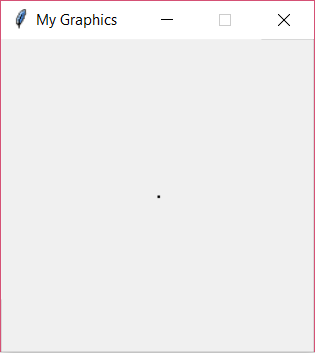### Circle

To create a Circle object, we need to specify the point of its center and the radius. To draw a circle in the middle of our above graphics window with radius 50 we can change the statement that draws the Point to create a Circle instead:

g = Circle(Point(125, 125), 50)

The graphics window will look at Figure 2 below: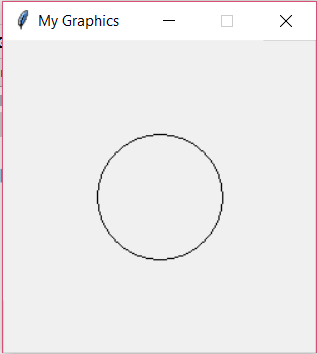All the objects can be customized such as setting interior color:

g.setFill("blue")

Or set outline color:

g.setOutline("red")

### Oval

An Oval object is formed by two points. For example:

g = Oval(Point(80, 100), Point(150, 150))

The graphics window will look at Figure 3 below: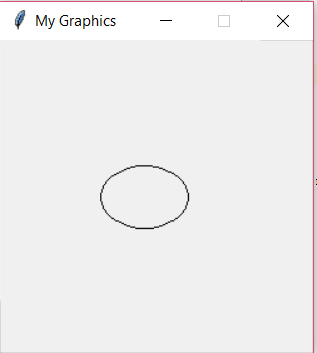The Oval object has a method to return the center of the Oval as Point:

c = g.getCenter()

We can get the X and Y coordinates for the Point:

print(c.getX(), c.getY())

And for the example above the values are: 115.0 125.0

### Line

A Line as you would expect also needs two Point objects. For example:

g = Line(Point(80, 100), Point(150, 150))

The graphics window will look at Figure 4 below: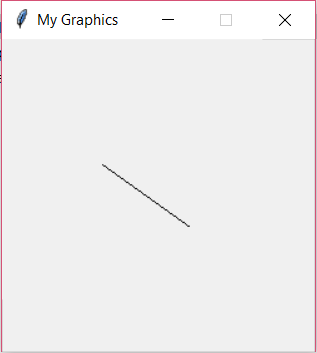You can also add an arrow to the line at either end:

g.setArrow("first")

The graphics window will look at Figure 5 below: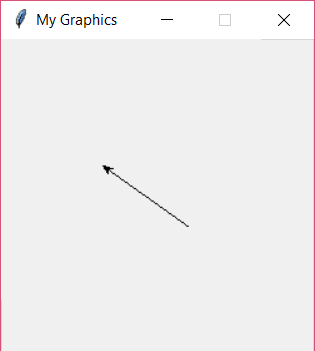As you can see the arrow is on the left side of the line. You can also put it on the end using the 'last' parameter or on both using the 'both' parameter.

You can also get back the Point in the middle of the line:

c = g.getCenter()

Which for the example above is: 115.0 125.0

### Text

We can also create a Text object giving it the anchor Point where the text starts and the text value:

To unlock this lesson you must be a Study.com Member.

### Register to view this lesson

Are you a student or a teacher?

### Unlock Your Education

#### See for yourself why 30 million people use Study.com

##### Become a Study.com member and start learning now.
Back
What teachers are saying about Study.com

### Earning College Credit

Did you know… We have over 160 college courses that prepare you to earn credit by exam that is accepted by over 1,500 colleges and universities. You can test out of the first two years of college and save thousands off your degree. Anyone can earn credit-by-exam regardless of age or education level.